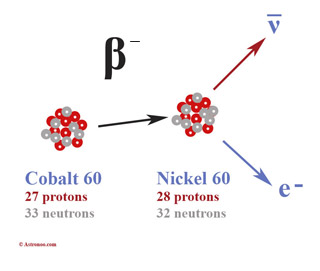Automatic translation Category: matter and particles
Updated April 4, 2015### Image: Four of these elementary particles would be sufficient in principle to build the world around us: the up and down quarks, the electron and the electron neutrino. The others are unstable and decay to reach these four particles. The sources of neutrinos in nature are the Earth, the atmosphere, the Sun, supernovae, nuclear reactors and humans via the few mg of radioactive potassium in it. A human being emits ≈340 million neutrinos per day.### Image: example of beta decay to the radioactive isotope cobalt 60 (60Co). Cobalt core 60, contains 33 neutrons (gray) and 27 protons (red), it has an excess of 6 neutrons. A neutron will be transformed into a proton. Cobalt core 60 becomes more stable and is transformed into nickel 60 (60Ni+) with 28 protons (1 more) and 32 neutrons (1 less), but still 60 nucleons. During the disintegration, two particles are created, an electron and an electronic antineutrino. There are two variants of beta decay, beta minus decay, as here with cobalt 60 which is transmuted into nickel 60 with the emission of an electron and beta plus decay that emits a positron and an electron neutrino.

The lepton is an elementary particle on which the strong nuclear force has no influence. The electron, positron and neutrino are low mass particles grouped under the name of lepton, which in Greek means [Light]. Leptons are fermions (building blocks of the material), that is, the spin 1/2 particles. Charged leptons are the electron and the positron, the muon and its anti-particulates, tauon, and its anti-particles. In the standard model of particle physics, there are twelve flavors of elementary fermions: six quarks and six leptons. Unlike quarks leptons interact through the strong interaction. The electronvolt (eV symbol) is a unit of energy or mass. Its value is defined as the kinetic energy gained by an electron accelerated from the repose by a difference potential of 1 volt. An electron volt is about 1 eV = 1.602 176 565 (35) × 10−19 joule (J). According to special relativity E = m · c2, we deduce: 1 eV / c2 = 1.783 × 10−36. The mass of the electron is 511 keV / c2, the proton 938 MeV / c2 and that the neutron is 940 MeV / c2. An electron is an elementary particle of the atom, it is a universal constituent of matter that has a negative electric charge sign denoted e. The electron has a charge considered indivisible, e = 1.59 x 10-19 coulomb and a mass m = 9 x 10-28 gramme. An electron volts, eV = 1.602 x 10−19 joule. The concept of an indivisible amount of electric charge is developed from 1838 by the British naturalist Richard Laming (1798  − 1879). The Greeks noticed that amber attracted small objects when rubbed with fur. The Greek word ἤλεκτρον (electron) refers to amber. The electron was the first elementary particle demonstrated in many experiments from the late nineteenth and early twentieth centuries. When the neutrino passes through matter, its property (flavor) is changed. The neutrino oscillation is a phenomenon of quantum mechanics, in which a neutrino appeared with some flavor lepton (electron, muon or tau) can later get a different flavor. Theoretically, a neutrino can oscillate in three flavors.
1997 © Astronoo.com − Astronomy, Astrophysics, Evolution and Earth science.Scale of the nanoparticles...Magnetic order
and magnetization...Discovery of buckyballs
solid in space...